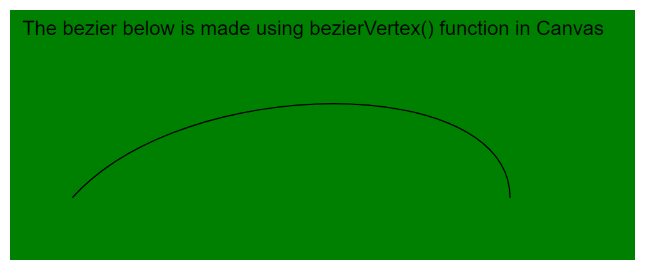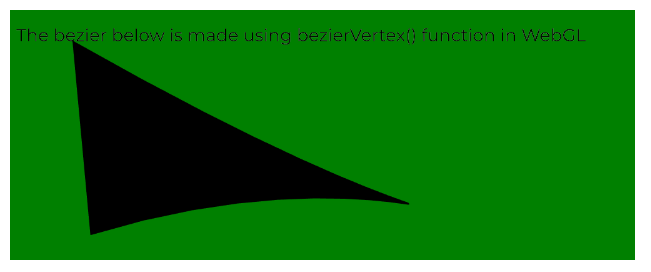# p5.js | bezierVertex() Function

• Last Updated : 25 May, 2020

The bezierVertex() function in p5.js is used to specify the vertex coordinates used to draw a bezier curve. Every call to this function defines the position of two control points and one anchor point of the bezier curve. This function can only be used between beginShape() and endShape(). It must be prefaced with a call to the vertex() function to set the first anchor point, when called with beginShape() for the first time.

The function expects 6 parameters in 2D mode and 9 parameters (which includes z coordinates) in 3D mode. Both the 2D and 3D modes can be used for drawing in the WebGL mode.

Syntax:

`bezierVertex( x2, y2, x3, y3, x4, y4 )`

OR

`bezierVertex( x2, y2, z2, x3, y3, z3, x4, y4, z4 )`

Parameters: This function accepts nine parameters as mentioned above and described below:

• x2: It is a number that specifies the x-coordinate of the first control point.
• y2: It is a number that specifies the y-coordinate of the first control point.
• x3: It is a number that specifies the x-coordinate of the second control point.
• y3: It is a number that specifies the y-coordinate of the second control point.
• x4: It is a number that specifies the x-coordinate of the anchor point.
• y4: It is a number that specifies the y-coordinate of the anchor point.
• z2: It is a number that specifies the z-coordinate of the first control point. It is used for the WebGL mode.
• z3: It is a number that specifies the z-coordinate of the second control point. It is used for the WebGL mode.
• z4: It is a number that specifies the z-coordinate of the anchor point. It is used for the WebGL mode.

The examples below illustrates the bezierVertex() function in p5.js:

Example 1:

 `function` `setup() {``  ``createCanvas(500, 200);``  ``textSize(16);``}`` ` `function` `draw() {``  ``background(``"green"``);``  ``fill(``"black"``);``  ``text(``"The bezier below is made using bezierVertex() function in Canvas"``, 10, 20);`` ` `  ``// Define the points``  ``// First Anchor Point``  ``let p1 = { x: 50, y: 150 };`` ` `  ``// First Control Point``  ``let p2 = { x: 140, y: 50 };`` ` `  ``// Second Control Point``  ``let p3 = { x: 400, y: 50 };`` ` `  ``// Anchor Point``  ``let p4 = { x: 400, y: 150 };`` ` `  ``noFill();``   ` `  ``// Start the bezier``  ``beginShape();`` ` `  ``// Specify the first anchor point``  ``vertex(p1.x, p1.y);`` ` `  ``// Specify the other points for bezierVertex()``  ``bezierVertex(p2.x, p2.y, p3.x, p3.y, p4.x, p4.y);``  ``endShape();``}`

Output:Example 2:

 `let newFont;`` ` `function` `preload() {``  ``newFont = loadFont(``"fonts/Montserrat.otf"``);``}`` ` `function` `setup() {``  ``createCanvas(500, 200, WEBGL);``  ``textFont(newFont, 14);``}`` ` `function` `draw() {``  ``background(``"green"``);``  ``fill(``"black"``);``  ``text(``"The bezier below is made using bezierVertex() function in WebGL"``, -245, -75);`` ` `  ``// Define the points``  ``// First Anchor Point``  ``let p1 = { x: -200, y: -75, z: 0 };`` ` `  ``// First Control Point``  ``let p2 = { x: 200, y: 150, z: 10 };`` ` `  ``// Second Control Point``  ``let p3 = { x: 100, y: -10, z: 10 };`` ` `  ``// Anchor Point``  ``let p4 = { x: -175, y: 75, z: 10 };`` ` `  ``noFill();`` ` `  ``// Start the bezier``  ``beginShape();`` ` `  ``// Specify the first anchor point``  ``vertex(p1.x, p1.y, p1.z);`` ` `  ``// Specify the other points for bezierVertex()``  ``bezierVertex(p2.x, p2.y, p2.z, p3.x, p3.y, p3.z, p4.x, p4.y, p4.z);``  ``endShape();``}`

Output:Online editor: https://editor.p5js.org/

My Personal Notes arrow_drop_up